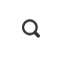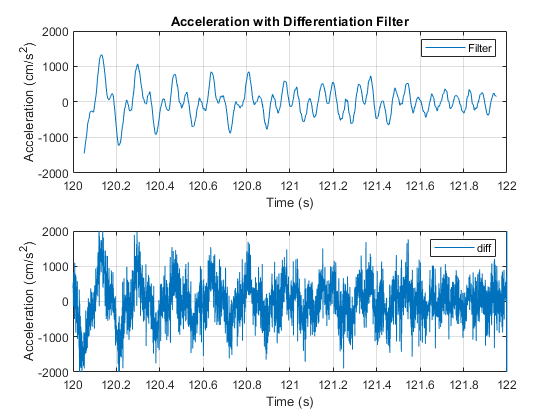Delta-Delta feature is proposed in 1986 by S. Furui and Hermann Ney in 1990. It’s simply add first and second derivative of cepstrum to the feature vector. By doing that they say it can capture spectral dynamics and improve overall accuracy.

The only problem is that in a discrete signal space getting derivative from the signal increase spontaneous noise level so instead of simple first and second order derivative HTK proposed a `differentiation filter`. This filter basically is a convoluted low-pass filter on top of discrete signal derivative to smooth out the result and remove unwanted noises. In Fig 1 you can see the result of simple second derivative vs the proposed `differentiation filter`.Fig. 1. Plain dervative VS differentiation filter. courtesy of Matlab™

HTK filter for a Delta-Delta feature (order=2, window=2) is a 9 element FIR filter with following coefficient(Θ is window size which is 2 in HTK)• Reverberation: Is the effect of sound bouncing the walls and getting back in a room. The time is roughly between 1 and 2 second in an ordinary room. You can use `Sabine equation` to do more accurate calculation.
• I’m thinking about using using `compressor filter` in speech instead of `CMVN` to normalize in real-time.
• The above formula c_t makes no sense. I will update this as soon as I can post codes here

IEEE ICASSP ’86 – Isolated Word Recognition Based on Emphasized Spectral Dynamics

IEEE ICASSP ’90 – Experiments on mixture-density phoneme-modelling for 1000-word DARPA task

Desh Raj Blog – Award-winning classic papers in ML and NLP

Recent Posts